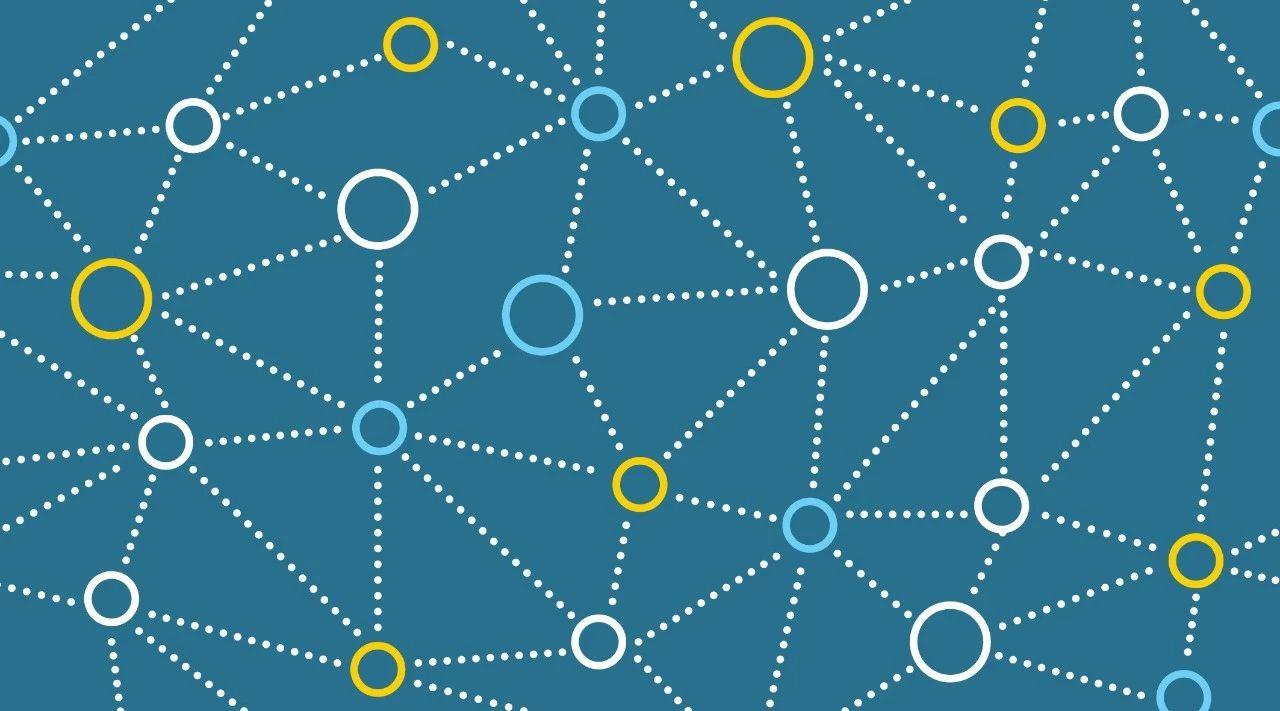Uber 利用卷积神经网络解决许多方面的问题，其中就包括坐标变换。从自动驾驶汽车的设计到路标的自动检测、再到地图的绘制，Uber 都需要使用卷积网络完成坐标变换。

CoordConv 层保留了前两个属性——参数较少和计算效率高，并且它的等变程度也可以学习。如果坐标中的权重学习为零，CoordConv 的表现将与标准卷积一样。另一方面，如果平移依赖对下游任务有用，那它也能学习这个。

CoordConv 涉及很多已有方法，如局部连接层、组合模式生成网络以及语言建模中使用的位置嵌入。以下展示了作者实现的核心代码，查看原论文可以了解有关此概念的更多讨论。

``````from tensorflow.python.layers import base
import tensorflow as tf

def __init__(self, x_dim=64, y_dim=64, with_r=False):
self.x_dim = x_dim
self.y_dim = y_dim
self.with_r = with_r

def call(self, input_tensor):
"""
input_tensor: (batch, x_dim, y_dim, c)
"""
batch_size_tensor = tf.shape(input_tensor)
xx_ones = tf.ones([batch_size_tensor, self.x_dim], dtype=tf.int32)
xx_ones = tf.expand_dims(xx_ones, -1)

xx_range = tf.tile(tf.expand_dims(tf.range(self.x_dim), 0), [batch_size_tensor, 1])
xx_range = tf.expand_dims(xx_range, 1)

xx_channel = tf.matmul(xx_ones, xx_range)
xx_channel = tf.expand_dims(xx_channel, -1)

yy_ones = tf.ones([batch_size_tensor, self.y_dim], dtype=tf.int32)
yy_ones = tf.expand_dims(yy_ones, 1)

yy_range = tf.tile(tf.expand_dims(tf.range(self.y_dim), 0), [batch_size_tensor, 1])
yy_range = tf.expand_dims(yy_range, -1)

yy_channel = tf.matmul(yy_range, yy_ones)
yy_channel = tf.expand_dims(yy_channel, -1)
xx_channel = tf.cast(xx_channel, ’float32’) / (self.x_dim - 1)
yy_channel = tf.cast(yy_channel, ’float32’) / (self.y_dim - 1)
xx_channel = xx_channel*2 - 1
yy_channel = yy_channel*2 - 1

ret = tf.concat([input_tensor, xx_channel, yy_channel], axis=-1)

if self.with_r:
rr = tf.sqrt( tf.square(xx_channel-0.5) + tf.square(yy_channel-0.5))
ret = tf.concat([ret, rr], axis=-1)
return ret

class CoordConv(base.Layer):
"""CoordConv layer as in the paper."""
def __init__(self, x_dim, y_dim, with_r, *args, **kwargs):
super(CoordConv, self).__init__()
self.conv = tf.layers.Conv2D(*args, **kwargs)

def call(self, input_tensor):
ret = self.conv(ret)
return ret``````

CoordConv 解决之前的监督任务

CoordConv 在多个领域中都能提供帮助